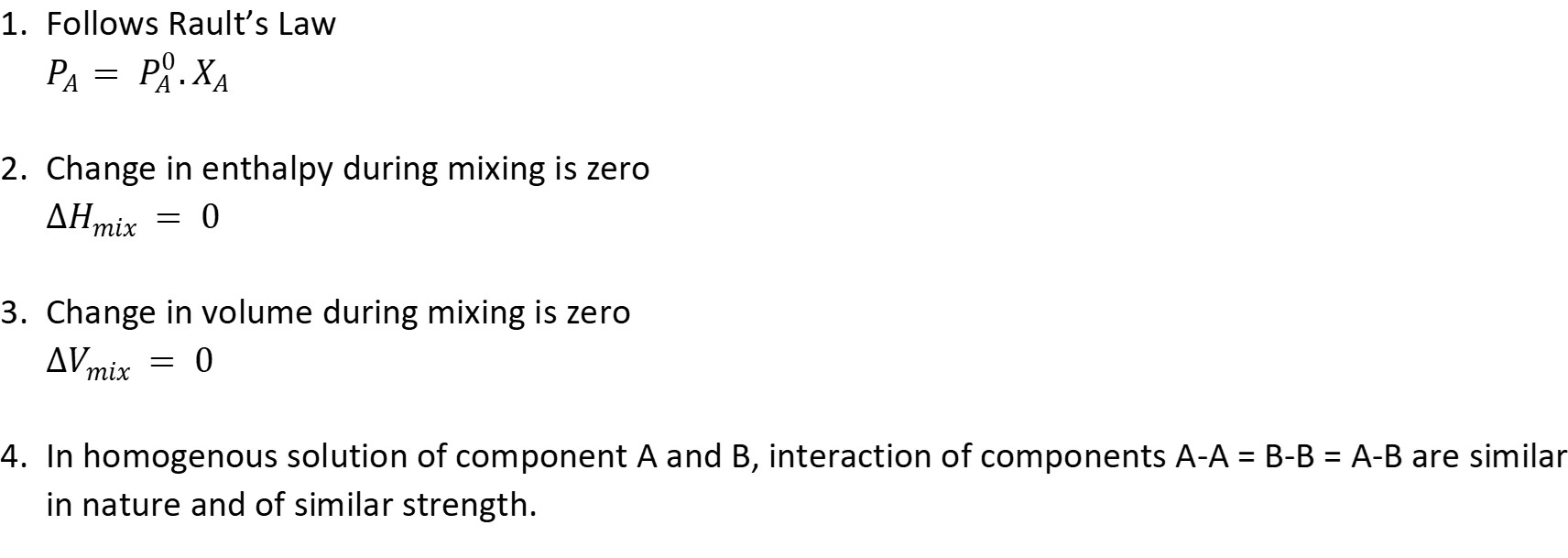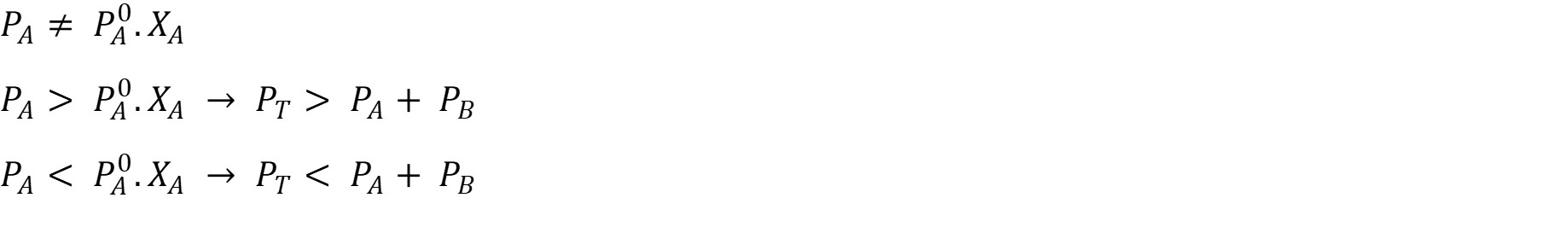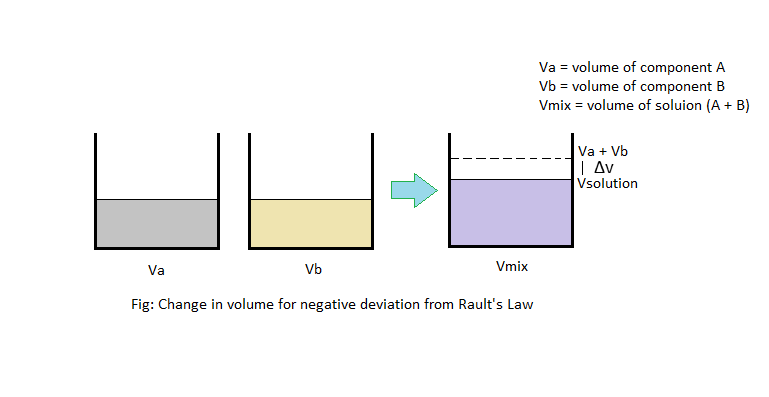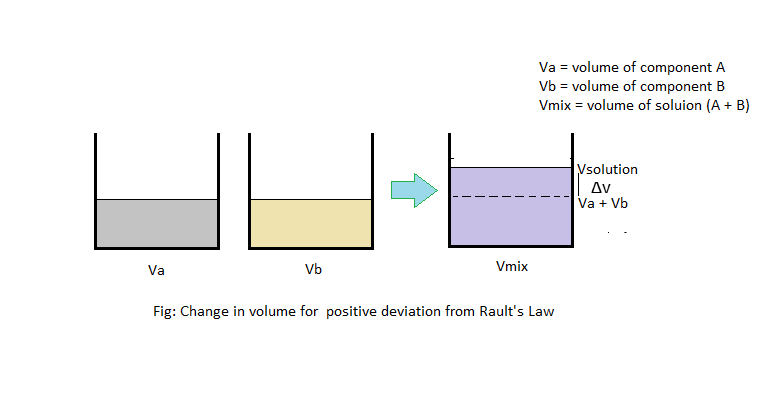# Ideal solution

Those solutions that follows Rault’s Law are called ideal solution. It exhibit following characteristics.Non-Ideal solution: Those solutions which do not follow Rault’s Law are called non ideal solutions.Negative deviation for Rault’s Law:When two components are  mixed together to form a homogeneous solution, the interaction between two components increases and as the result, molecules are bound close to each other, decreasing its volume. That means change in volume is negative. I.e. ∆Vmix < 0

Since the system have gain more stability, more energy must have been released, decreasing its enthalpy. I.e. ∆Hmix < 0

Also because of strong interaction between molecules of two components ad loss of enthalpy, components are less likely to go to vapor phase. Thus vapor pressure is less than that of ideal case. This can be graphically represented as following.

Positive deviation for Rault’s Law:When two components A and B are mixed together to form homogenous solution, the interaction between two components is very low as compared to ideal solution. Particles of two components are far from each other thereby increasing volume than in ideal solution. Thus change in volume is positive. I.e. ∆Vmix > 0.

Since the particles are far away from each other, the stability is reduced, indicating there must be absorption of some energy, increasing its enthalpy. I.e. ∆Hmix > 0

Again, since particles are far from each other, particles of individual components can easily find way to vaporize leading to increase in vaporization. Thus vapor pressure is more than that in ideal case.